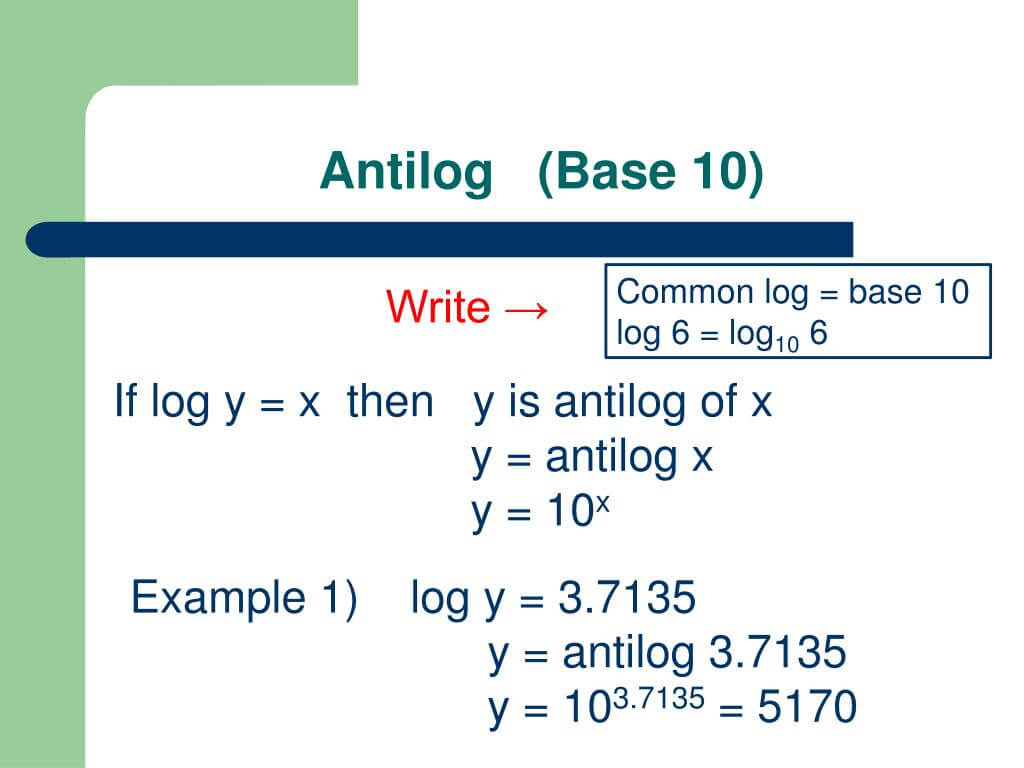# Antilog Calculator

Input the value and base of antilog, click the calculate button to find the antilog of a number using the antilog calculator.

Formula Antilog a (loga (x)) = x## Antilog Formula

Antilog a (log a (x)) = x

Antilog calculator is used to calculate the antilog of a number with the arbitrary base. It can calculate the antilog value with any given base. The inverse log calculator finds the inverse function of log with the specified base number. In this content, we will explain antilog, how to calculate antilog on calculator, what is the inverse of log 10, and much more.

## How to use our antilog calculator?

To calculate the antilog using the inverse of log base 10 calculator, follow these steps:

• Enter the antilog value in the given input box.
• Enter the antilog base in the next input box.
• Press the Calculate button to see the antilog.
• Press the Reset button to reset all values for a new calculation.

Antilog calculator instantly calculates the antilog value for the given number and base. To calculate the log of any number, you can use our log calculator anytime.

## What is antilog?

The logarithm x and base are known in some problems, but x is unknown. The opposite or inverse function of a logarithm is an antilogarithm. An antilog base is always a positive number because the root of an exponential function is not negative. Since the inverse is an exponential function of a logarithm,

Antilog a (log a (x)) = x

If log a x=b, then x is called the antilogarithm of b and is written as:

x = antilog a b = ab

The antilogarithm of b with base a is consequently ab. If the antilog basis isn't written, the base of the antilog will be 10b by default since log x means base 10 logarithm.## How to find antilog?

When the numbers are written in billions and trillions, it is relatively complicated to deal with those numbers. The log will work for you, irrespective of whether it concerns income, population growth, or large distances. It can make it easier to understand the large amounts of lengthy and complicated equations.

Antilog is used to reverse the log function in any number. We use antilog to get back the original number that we have acquired after using the logarithm.

To find antilog of a number with a base, follow these steps:

1. Write down the number to calculate the antilog.
2. Specify the base of the number to calculate antilog.
3. Raise the antilog number to the power of base b.
4. The resulting number will be the antilog number with the specified base.

Example:

Let's understand the antilog calculation by using an example.

Calculate antilog of 2 with the base 10.

Solution:

Step 1: Write down the number as a to calculate the antilog.

a = 2

Step 2: Specify the base of the number to calculate antilog.

b = 10

Step 3: Raise the antilog number to the power of base b.

x = antilog 2 10 = 2 10

x = 2 10 = 100

Step 4: Resulting number will be the antilog number with the specified base.

So, the antilog of 2 with base 10 is 100. Similarly, you can find the antilog of any number by using the above method.

## Some important antilog values

 antilog(2) 102 100 antilog(1) 101 10 antilog(10) 1010 1e+10 antilog2 5 25 32 antilog2 2 22 4 antilog(3) 103 1000 antilog3 5.5 35.5 420.888 antilog2 1.5 21.5 2.8284 antilog(15.6) 1015.6 3.98107e+15 antilog(8) 108 1e+08 antilog(0) 100 1 antilog(4) 104 10000 antilog(5) 105 100000 antilog(9) 109 1e+09 antilog(12) 1012 1e+12 antilog(20) 1020 1e+20 antilog(22) 1022 1e+22 antilog(13) 1013 1e+13 antilog(18) 1018 1e+18 antilog(5) 105 100000 antilog(14) 1014 1e+14